# Magical Maths

Math is magical but beyond it is Logical which makes math a Fun.
Here is one mathematical series, would you like to guess the logic for it.

0 1 1 2 3 5 8 13 21 . . . .

I think its quite easy to crack, so here is the logic: the next number is found by adding the two numbers before it.

0
1
1 (0 + 1)
2 (1 + 1)
3 (1 + 2)
5 (2 + 3)
8 (3 + 5)
and so on!

And we all know that this series or sequence is popular as Fibonacci Sequence. It is named after Italian Mathematician Leonardo Pisano Bogollo, his nickname was Fibonacci.
The numbers in Fibonacci Sequence are known as Fibonacci Numbers.

Let

n be the number upto which we want to write the fibinacci sequence.

Xn be the Fibonacci number in the sequence

So here we go for fibonacci sequence upto n = 5

n 0 1 2 3 4 5
Xn 0 1 1 2 3 5
Logic 0 1 X0 + X1 X1 + X2 X2 + X3 X3 + X4

From above table we can clearly write :

Xn = Xn-2 + Xn-1
Where
Xn = Fibonacci Number “n”
Xn-2 = Fibonacci Number “n-2”
Xn-1 = Fibonacci Number “n-1”

Here are the Squares representing a fibbonacci sequence upto n=5.

for n = 0 we have X0 = 0 thus there is no square for it, for n = 1 we have X1 = 1 and thus represented by a unit square and thus X3 = 2 represented by 4 (2 x 2) unit square and so on!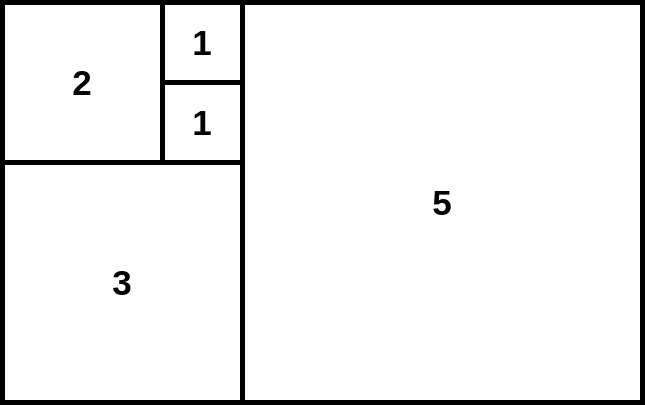The above representation is made using 8 x 5 unit squares and interestingly we are going to use it as a display of our clock. But How?

### References

2 Likes

The inspiration for making this clock came from a project on Kickstarter, here is the video:

The creator of this clock is Philippe Chrétien and named this Geek Clock as a Fibonacci Clock.
Building this clock is full of fun and required some maths to develop algorithm for clock to work as well as during reading time.
So what do you think about this geek clock and would you like to tell us what all components required to build it.

Stay tuned for development ahead. Happy Making !

3 Likes

Below are some images from my Build-in Progress so far which only include the display part and lighting the LEDs. BTW do you know what kind of LEDs i am using here.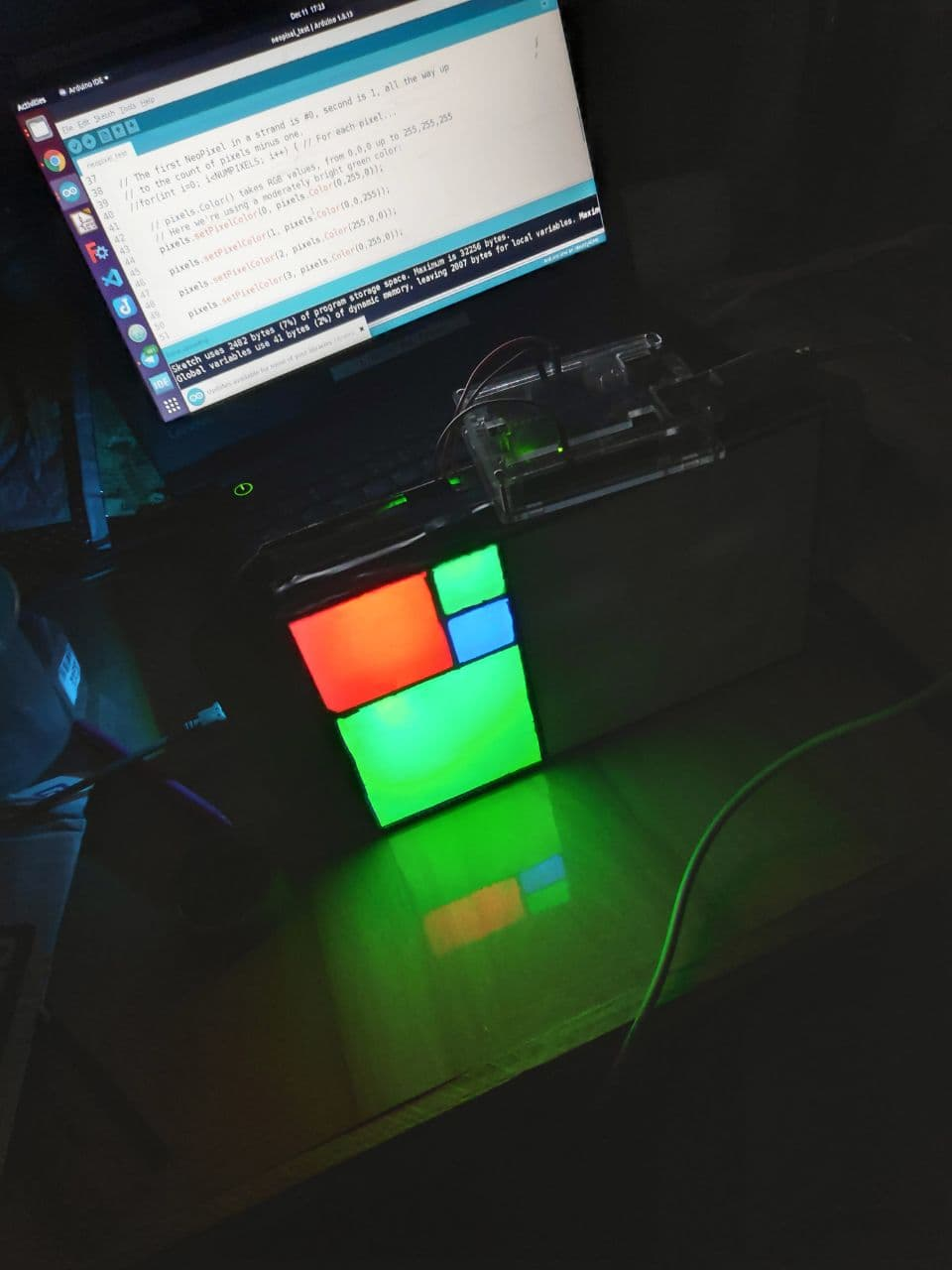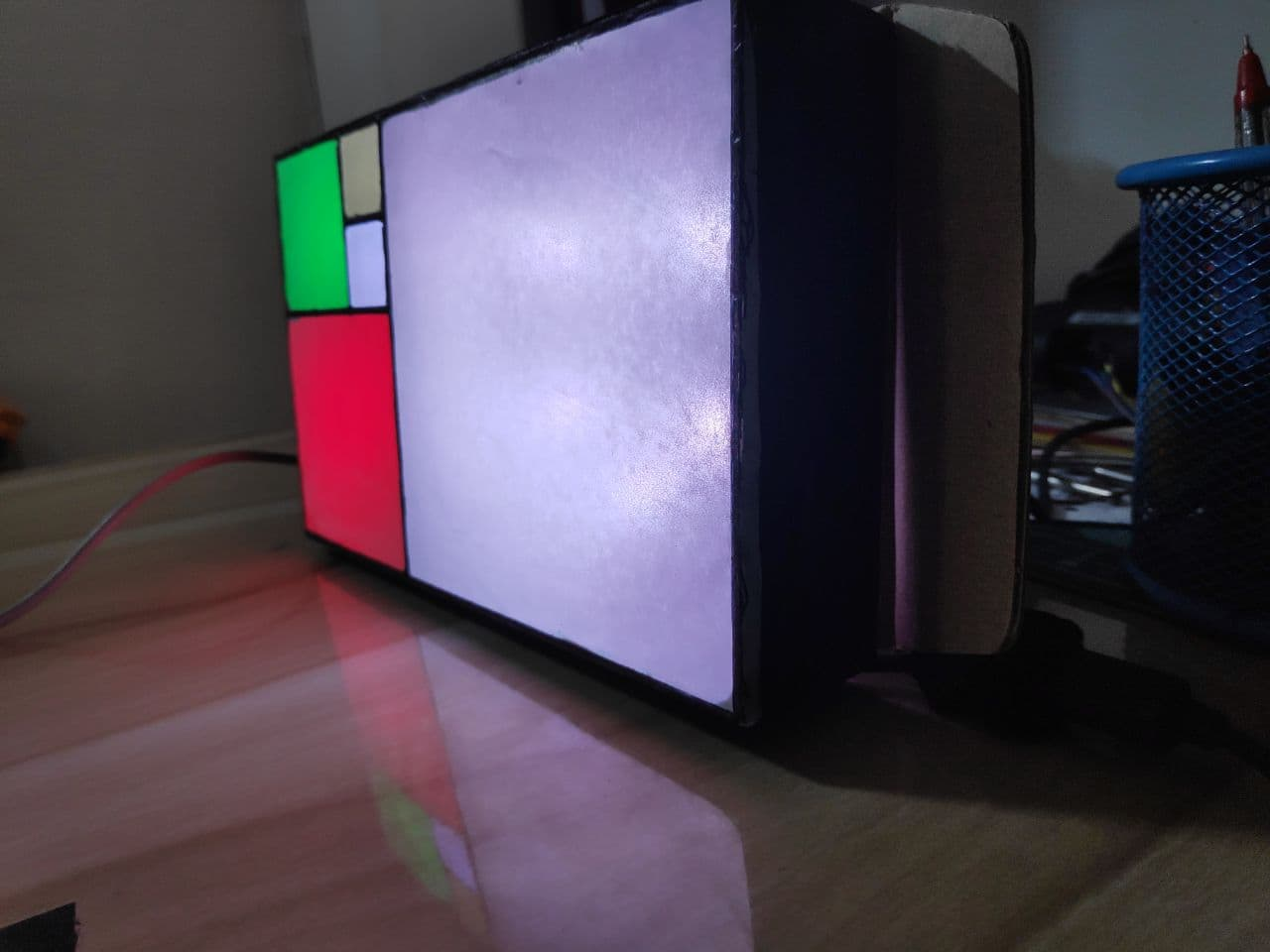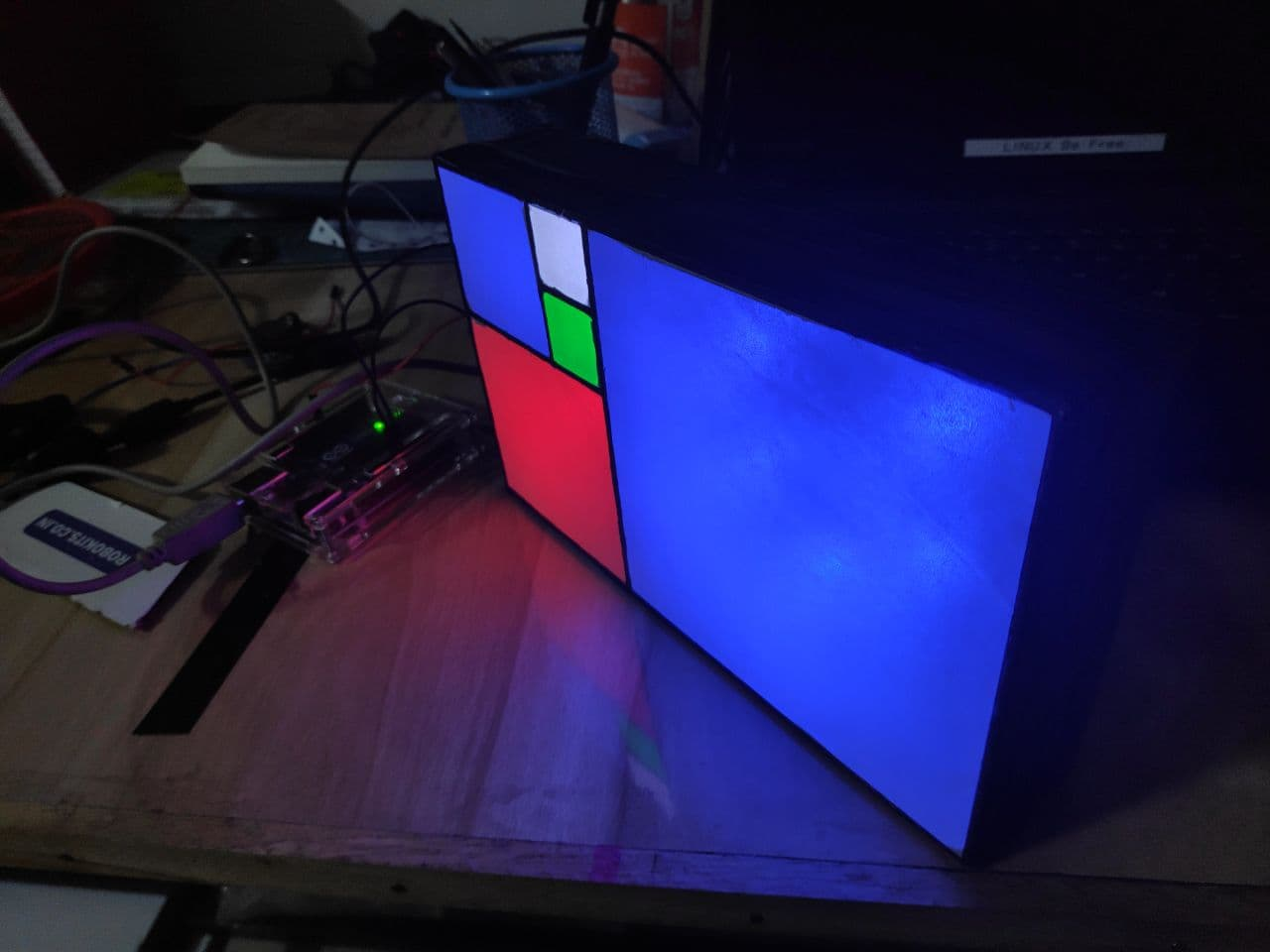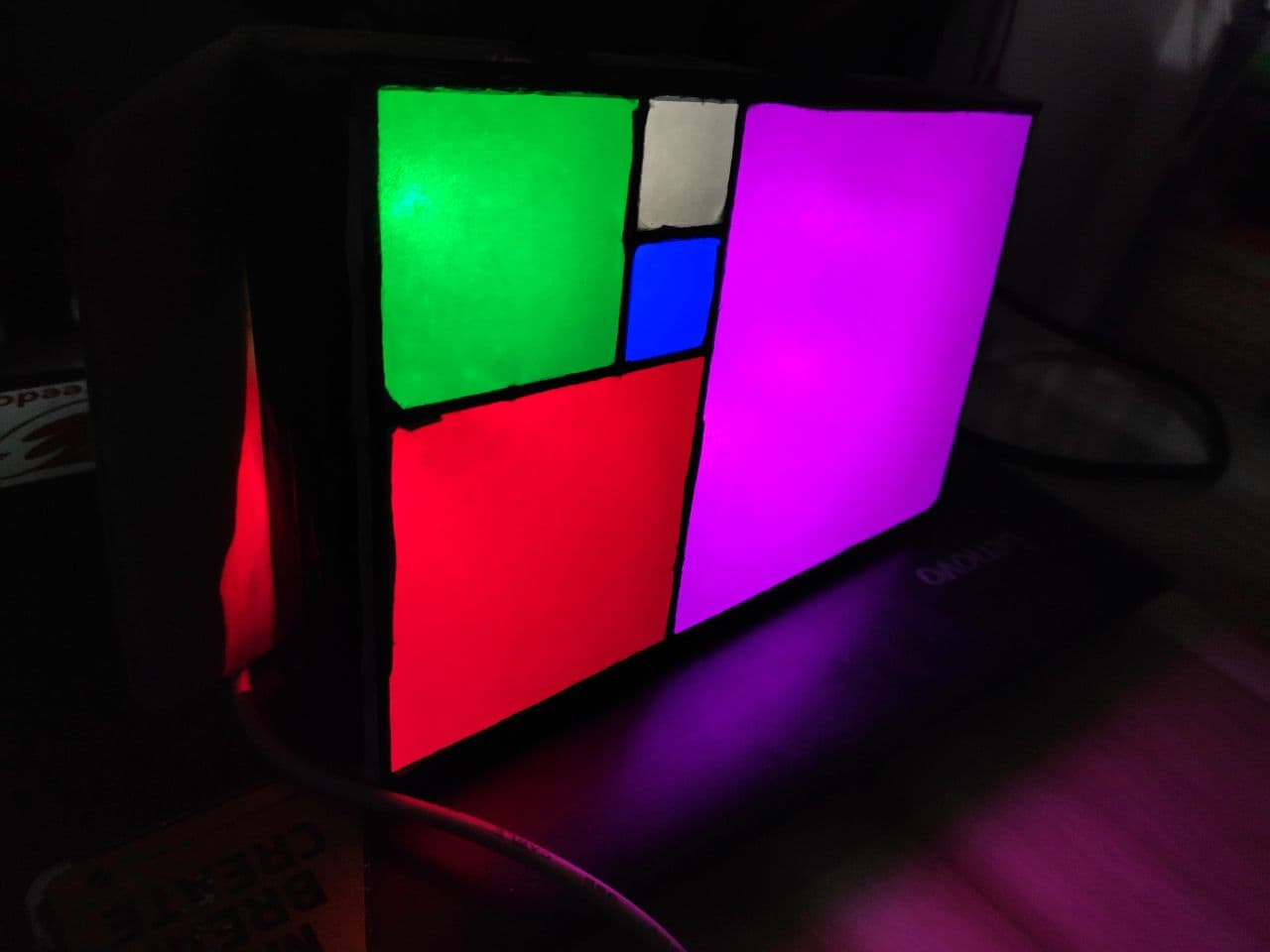Making Circuit and Writing a code for it is still pending, Will soon update the work done right from scratch. Mean While help to draw a Circuit Schematic for this clock with the features you want.

Stay Tuned, Happy Making!

2 Likes